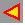# 3. Gaussian and Mean Curvatures at the Regular Point of a Ruled Surface

A ruled surface is a surface generated by a straight line moving along a curve. The straight lines themselves are called rulings.
The rulings of a ruled surface F are asymptotic curves.
The Gaussian curvature at a regular point of a ruled surface is nonpositive.

A ruling is torsal if a surface is touched along it by unique tangent plane. The points on a torsal ruling are parabolic.
A ruling is scrolar if a surface has a different tangent plane at each point on it. The points on a scrolar ruling are hyperbolic.

A ruled surface is developable if all its rulings are torsal. It can be projected onto a plane without stretching or shrinking any element. For such a surface the Gaussian curvature vanishes everywhere - all points are parabolic (tangent developables, cones, cylinders).
A ruled surface with a finite number of torsal lines are called a scroll. If a regular point of such a surface does not lie on its torsal ruling, it is a hyperbolic point.
If a scroll is a quadric (the hyperbolic paraboloid or the hyperboloid of one sheet) it is a double generated ruled surface - through each point on the surface two rulings pass. The rulings define the asimptotic directions.
All other algebraic ruled surfaces are simple generated - through each regular point on it exactly one ruling passes (3rd degree algebraic scrolls, 4th degree algebraic scrolls). The asimptotic directions at regular points of such a scroll are defined by the rulings and the tangent lines of the intersection curves of tangent planes and a scroll. For n-order algebraic ruled surfaces these intersections are (n-1)-order algebraic curves.
At a regular point the principal directions bisect the angle between the asymptotic directions.

3.1 EXAMPLE (the hyperbolic paraboloid)

3.2 EXAMPLE (the 4th degree conoid)back